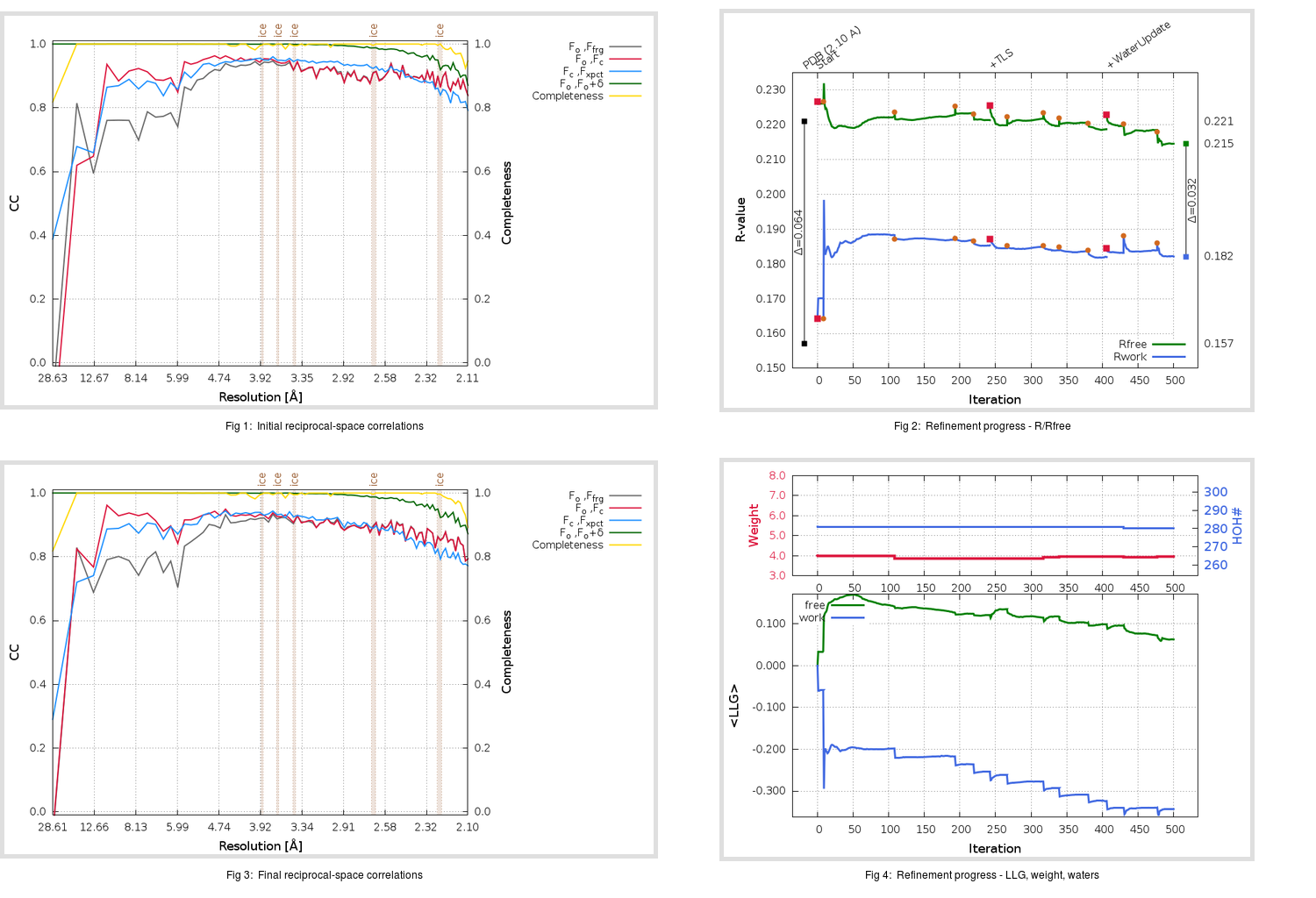Content:

## Deposited

` `
 Date deposited Date data collection Resolution R, Rfree 20200316 20200215 2.10 0.1500 0.2210

Molprobity (CCP4 7.0 version) summary:

```Ramachandran outliers =   0.33 %
favored =  97.69 %
Rotamer outliers      =   4.96 %
C-beta deviations     =     0
Clashscore            =   4.04
RMS(bonds)            =   0.0109
RMS(angles)           =   1.39
MolProbity score      =   1.79
Resolution            =   2.10
R-work                =   0.1500
R-free                =   0.2210
```

```Number of waters      =   281

<B> (all atoms) =   30.78 ( sd =   14.02 ) for       2673 non-hydrogen atoms
<B>   (protein) =   29.92 ( sd =   14.32 ) for       2358 non-hydrogen atoms
<B>     (water) =   38.48 ( sd =    8.97 ) for        281 non-hydrogen atoms
<B>    (others) =   27.39 ( sd =    4.32 ) for         34 non-hydrogen atoms

B min/max       (all non-hydrogen atoms) =   10.07 /  132.58
B min/max   (protein non-hydrogen atoms) =   10.07 /  132.58
B min/max     (water non-hydrogen atoms) =   13.03 /   58.16
B min/max     (other non-hydrogen atoms) =   20.17 /   36.31
```

## BUSTER (re-)refinement

` `

Molprobity (CCP4 7.0 version) summary:

```Ramachandran outliers =   0.33 %
favored =  98.02 %
Rotamer outliers      =   3.05 %
C-beta deviations     =     0
Clashscore            =   1.06
RMS(bonds)            =   0.0112
RMS(angles)           =   1.57
MolProbity score      =   1.18
Resolution            =   2.10
R-work                =   0.1821
R-free                =   0.2146
```

```Number of waters      =   280

<B> (all atoms) =   28.47 ( sd =    9.84 ) for       2672 non-hydrogen atoms
<B>   (protein) =   27.05 ( sd =    8.66 ) for       2358 non-hydrogen atoms
<B>     (water) =   40.97 ( sd =   11.05 ) for        280 non-hydrogen atoms
<B>    (others) =   25.19 ( sd =    1.43 ) for         34 non-hydrogen atoms

B min/max       (all non-hydrogen atoms) =   12.69 /   70.96
B min/max   (protein non-hydrogen atoms) =   13.81 /   69.72
B min/max     (water non-hydrogen atoms) =   12.69 /   70.96
B min/max     (other non-hydrogen atoms) =   22.86 /   27.86
```

Refinement progression:Results:

` `
 File Remark 6W63_aB_refine.01_03_refine.pdb.gz exact refinement commands are in header 6W63_aB_refine.01_03_refine.mtz.gz including original deposited data and several re-refinement map coefficients 6W63_aB_refine.01_03_BUSTER_model.cif.gz including any non-standard compound restraints 6W63_aB_refine.01_03_BUSTER_refln.cif.gz